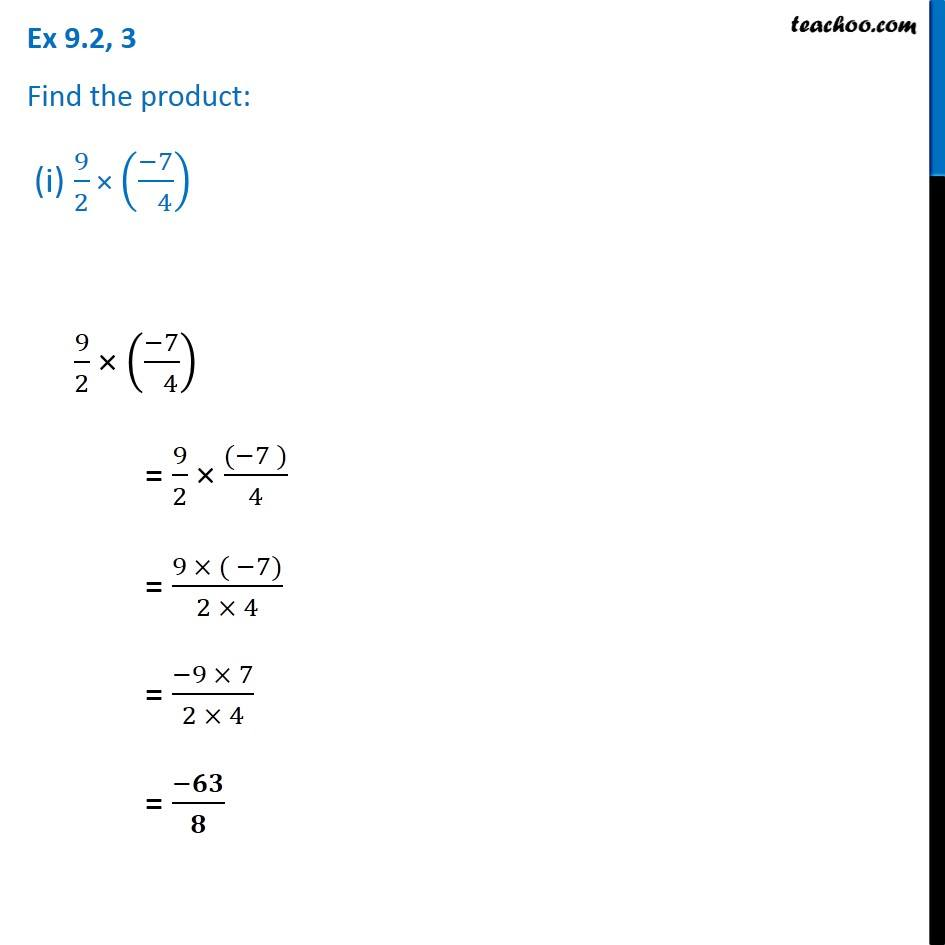Multiplication of rational numbers

Chapter 9 Class 7 Rational Numbers
Concept wiseIntroducing your new favourite teacher - Teachoo Black, at only ₹83 per month

### Transcript

Ex 9.2, 3 Find the product: (i) 9/2 × ((−7)/( 4)) 9/2 × ((−7)/( 4)) = 9/2 × ((−7 ))/4 = (9 × ( −7))/(2 × 4) = (−9 × 7)/(2 × 4) = (−𝟔𝟑)/𝟖